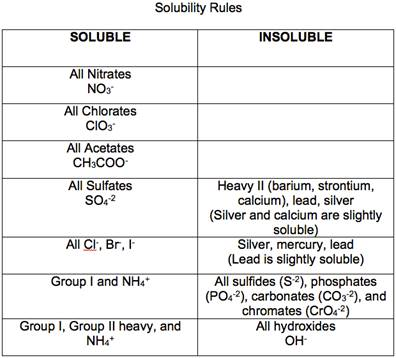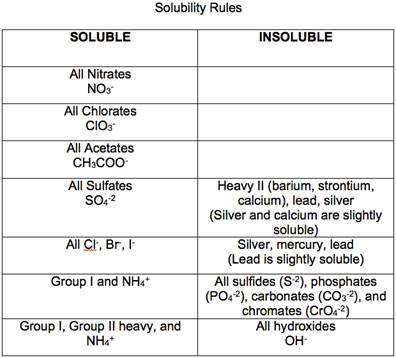Chapter 17, Problem 40PS

Chapter
Section
Textbook Problem

Predict whether each of the fallowing is insoluble or soluble in water.(a) Pb(NO3)2(b) Fe(OH)3(c) ZnCl2(d) CuS

a)

Interpretation Introduction

Interpretation:

The following ion (NH4)2CO3 is insoluble or soluble in water by using the solubility gude lines has to be determined.

Concept introduction:

Precipitation reactions are exchange reactions in which one of the products is a water - insoluble compounds.

For example -CaCO3

CaCl2(aq) + Na2CO3(aq)  CaCO3(s) + 2NaCl(aq)

The solubility of a compound is defined as the amount of that substance that can be dissolved in a given quantity of a solvent.

All ionic compounds of alkali metals and ammonium ions are soluble in water.Explanation

(NH4)2

b)

Interpretation Introduction

Interpretation:

The following ion Fe(OH)3 is insoluble or soluble in water by using the solubility gude lines has to be determined.

Concept introduction:

Precipitation reactions are exchange reactions in which one of the products is a water - insoluble compounds.

For example -CaCO3

CaCl2(aq) + Na2CO3(aq)  CaCO3(s) + 2NaCl(aq)

The solubility of a compound is defined as the amount of that substance that can be dissolved in a given quantity of a solvent.

All ionic compounds of alkali metals and ammonium ions are soluble in water.c)

Interpretation Introduction

Interpretation:

The following ion ZnCl2 is insoluble or soluble in water by using the solubility gude lines has to be determined.

Concept introduction:

Precipitation reactions are exchange reactions in which one of the products is a water - insoluble compounds.

For example -CaCO3

CaCl2(aq) + Na2CO3(aq)  CaCO3(s) + 2NaCl(aq)

The solubility of a compound is defined as the amount of that substance that can be dissolved in a given quantity of a solvent.

All ionic compounds of alkali metals and ammonium ions are soluble in water.d)

Interpretation Introduction

Interpretation:

The following ion CuS is insoluble or soluble in water by using the solubility gude lines has to be determined.

Concept introduction:

Precipitation reactions are exchange reactions in which one of the products is a water - insoluble compounds.

For example -CaCO3

CaCl2(aq) + Na2CO3(aq)  CaCO3(s) + 2NaCl(aq)

The solubility of a compound is defined as the amount of that substance that can be dissolved in a given quantity of a solvent.

All ionic compounds of alkali metals and ammonium ions are soluble in water.Still sussing out bartleby?

Check out a sample textbook solution.

See a sample solution

The Solution to Your Study Problems

Bartleby provides explanations to thousands of textbook problems written by our experts, many with advanced degrees!

Get Started

Why are significant figures used?

An Introduction to Physical Science

What does the word apparent mean in apparent visual magnitude?

Horizons: Exploring the Universe (MindTap Course List)

What is the role of the cytochromes in the electron transport chain?

Chemistry for Today: General, Organic, and Biochemistry